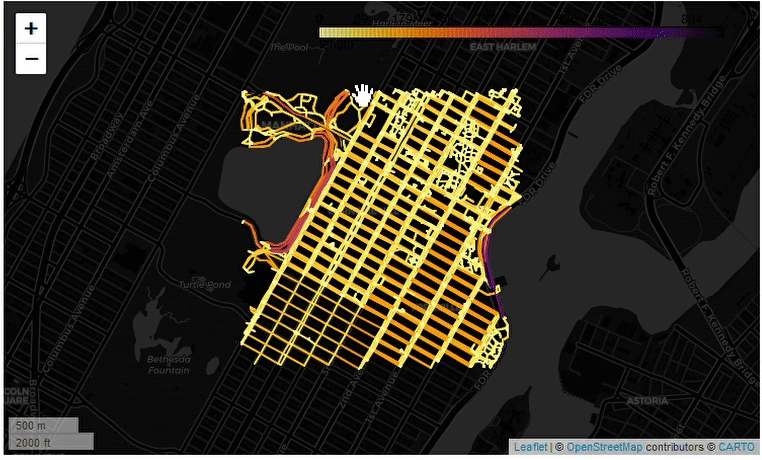# Exploring geospatial data in Python with GeoPandas

GeoPandas, is a python library that extends the data types used by Pandas to allow spatial operations on geometric types. Facilitating to extend traditional workflows to include geospatial data, and make spatial operation in the data using shapely.

One of the first tasks when working with data is to inspect it to have a better understanding of what are we dealing with. Whit geospatial data this exploration can involve displaying the data in a map, to better understand how it extends. Using GeoPandas this exploration is straightforward.

Before getting into the details on how to do it, we need to have some geospatial data to play with. In this case I’ll be using data from OpenStreetMap, downloading it with OSMnx (Examples of OSMnx for analyzing urban topology, and amenities), the data can be collected with the following code:

import osmnx as ox

G = ox.graph_from_address('Manhattan, New York')


The previous code will return a Networkx graph from the specified address. Now the task of exploring the data inside it. OSMnx has a function to extract geopandas.GeoDataFrame from the networks, we can get the nodes and edges with:

node, edges = ox.graph_to_gdfs(G)


Now, using the .explore() method from geopandas.GeoDataFrame we can explore the data from the edges with the following code:

edges.explore(column = "length",
tooltip = "length",
popup = True,
tiles = "CartoDB dark_matter",
cmap = "inferno_r"
)


The method .explore() on the edges takes different parameters to customize how the data will be displayed, the column parameter takes the name of the column used to set the color, tooltip sets the column to be displayed when hovering over the data, popup controls if we want to show a popup with the data set in the tooltip, and finally, the tiles controls the background tiles, and the cmap the color map used. The result:In case we want to save the map into an HTML file, we can do it with:

edges_map = edges.explore(column = "length",
tooltip = "length",
popup = True,
tiles = "CartoDB dark_matter",
cmap = "inferno_r"
)

output_file_path = "edges_manhattan.html"

edges_map.save(out_file_path)


### 💬 Join the conversation:

Keep to conversation with a comment or reach out in my social networks